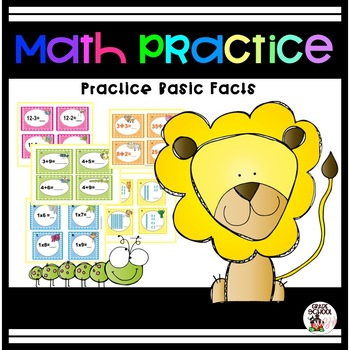# Math Practice;
K - 3rd
Subjects
Resource Type
Standards
Formats Included
• Zip
\$10.32
List Price:
\$14.75
You Save:
\$4.43
Bundle
\$10.32
List Price:
\$14.75
You Save:
\$4.43
Bundle
Report this resource to TPT

### Description

Math practice provides task cards for a variety of math facts. It will provide your students with addition facts, subtraction facts, multiplication facts, division facts, and tens and ones. It will take you through the whole year. Also they can be used in centers or for fast finishers.

Total Pages
N/A
Teaching Duration
N/A
Report this resource to TPT
Reported resources will be reviewed by our team. Report this resource to let us know if this resource violates TPT’s content guidelines.

### Standards

to see state-specific standards (only available in the US).
Compose and decompose numbers from 11 to 19 into ten ones and some further ones, e.g., by using objects or drawings, and record each composition or decomposition by a drawing or equation (e.g., 18 = 10 + 8); understand that these numbers are composed of ten ones and one, two, three, four, five, six, seven, eight, or nine ones.
Understand that the two digits of a two-digit number represent amounts of tens and ones. Understand the following as special cases:
Add within 100, including adding a two-digit number and a one-digit number, and adding a two-digit number and a multiple of 10, using concrete models or drawings and strategies based on place value, properties of operations, and/or the relationship between addition and subtraction; relate the strategy to a written method and explain the reasoning used. Understand that in adding two-digit numbers, one adds tens and tens, ones and ones; and sometimes it is necessary to compose a ten.
Fluently add and subtract within 100 using strategies based on place value, properties of operations, and/or the relationship between addition and subtraction.
Fluently add and subtract within 5.